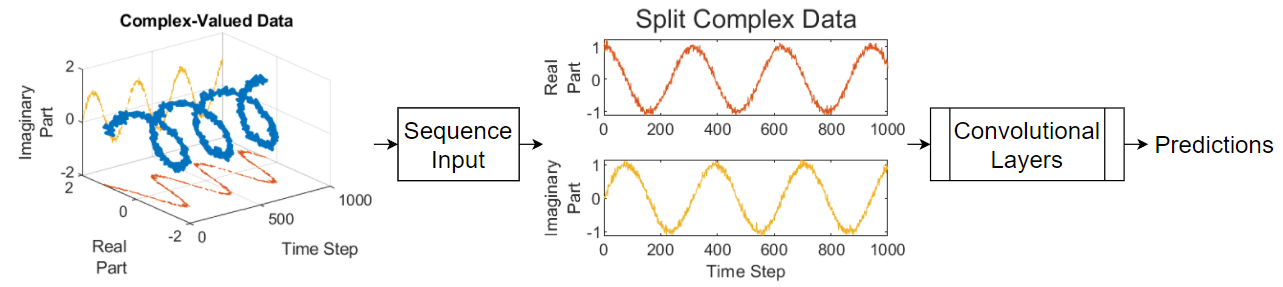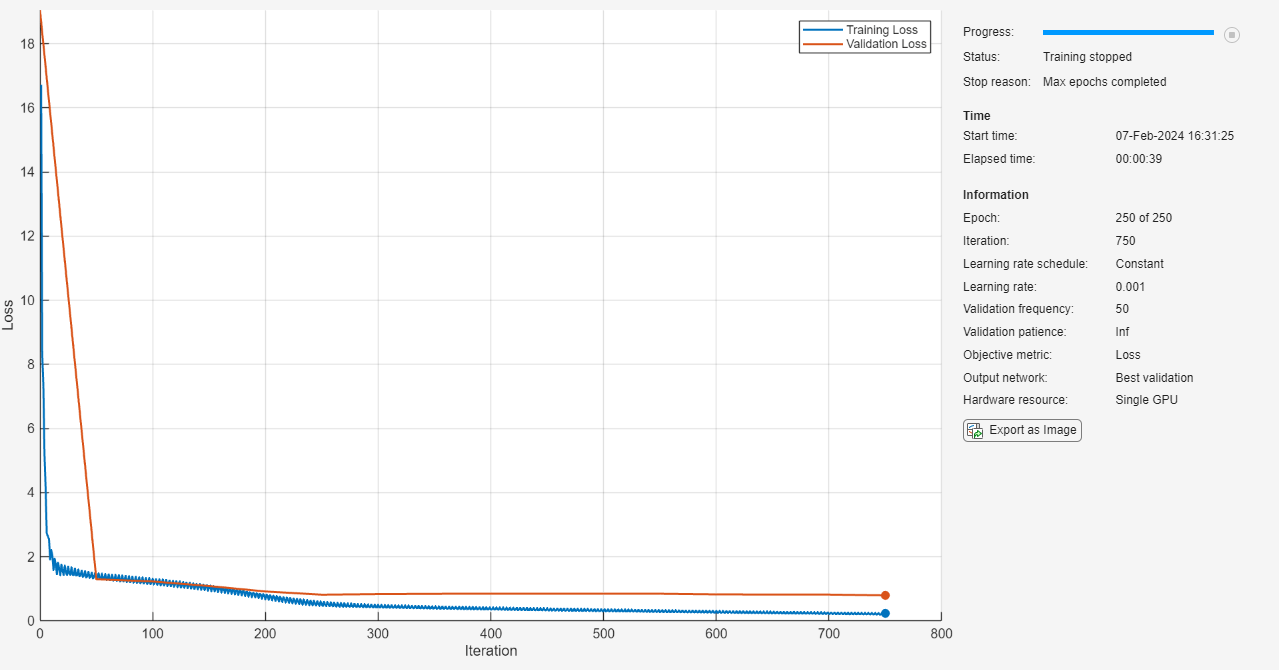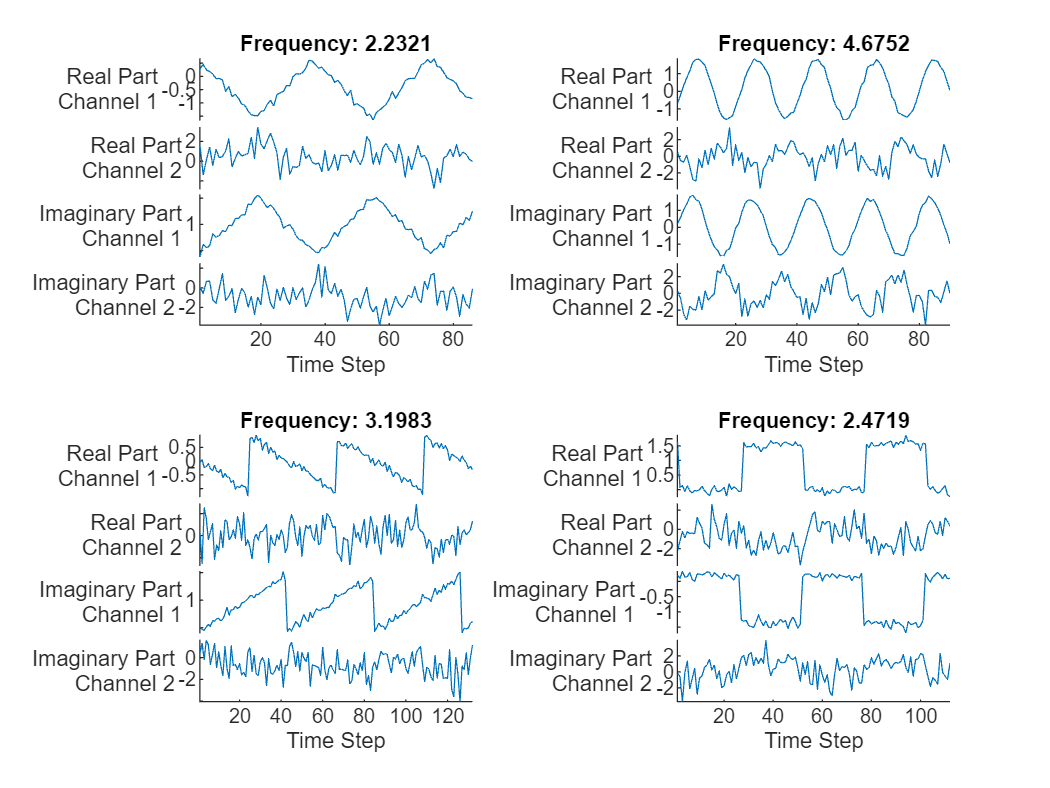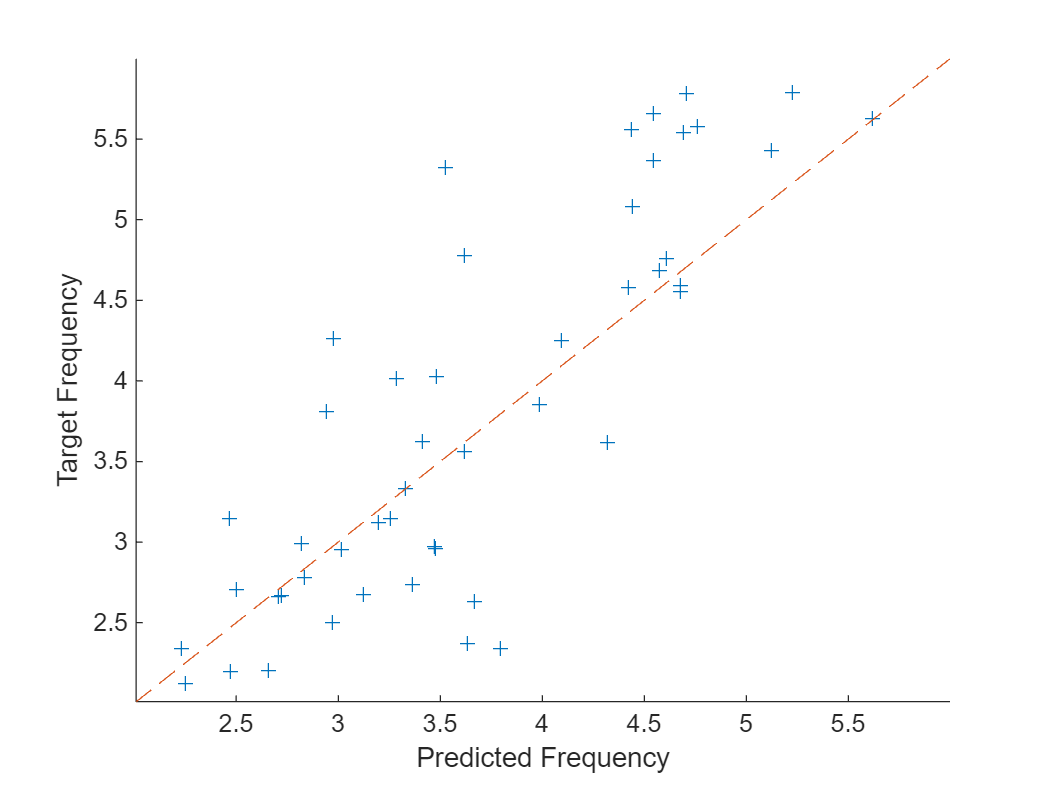# Train Network with Complex-Valued Data

This example shows how to predict the frequency of a complex-valued waveform using a 1-D convolutional neural network.

To pass complex-valued data to a neural network, you can use the input layer to split the complex values into their real and imaginary parts before it passes the data to the subsequent layers in the network. When the input layer splits the input data in this way, the layer outputs the split data as extra channels. This diagram shows how complex-valued data flows through a convolutional neural network.To split the complex-valued data into its real and imaginary parts as its input to a network, set the `SplitComplexInputs` option of the network input layer to `1` (`true`).

This example trains a sequence-to-one regression network using the Complex Waveform data set, which contains 500 synthetically generated complex-valued waveforms of varying lengths with two channels. The network trained in this example predicts the frequency of the waveforms.

Load the example data from `ComplexWaveformData.mat`. The data is a `numObservations`-by-1 cell array of sequences, where `numObservations` is the number of sequences. Each sequence is a `numChannels`-by-`numTimeSteps` complex-valued array, where `numChannels` is the number of channels of the sequence and `numTimeSteps` is the number of time steps in the sequence. The corresponding targets are in a `numObservations`-by-`numResponses` numeric array of the frequencies of the waveforms, where `numResponses` is the number of channels of the targets.

`load ComplexWaveformData`

View the number of observations.

`numObservations = numel(data)`
```numObservations = 500 ```

View the sizes of the first few sequences and the corresponding frequencies.

`data(1:4)`
```ans=4×1 cell array {2×157 double} {2×112 double} {2×102 double} {2×146 double} ```
`freq(1:4,:)`
```ans = 4×1 5.6232 2.1981 4.6921 4.5805 ```

View the number of channels of the sequences. For network training, each sequence must have the same number of channels.

`numChannels = size(data{1},1)`
```numChannels = 2 ```

View the number of responses (the number of channels of the targets).

`numResponses = size(freq,2)`
```numResponses = 1 ```

Visualize the first few sequences in plots.

```displayLabels = [ ... "Real Part" + newline + "Channel " + string(1:numChannels), ... "Imaginary Part" + newline + "Channel " + string(1:numChannels)]; figure tiledlayout(2,2) for i = 1:4 nexttile stackedplot([real(data{i}') imag(data{i}')],DisplayLabels=displayLabels) xlabel("Time Step") title("Frequency: " + freq(i)) end```### Prepare Data for Training

Set aside data for validation and testing. Partition the data into a training set containing 80% of the data, a validation set containing 10% of the data, and a test set containing the remaining 10% of the data. To partition the data, use the `trainingPartitions` function, attached to this example as a supporting file. To access this file, open the example as a live script.

```[idxTrain,idxValidation,idxTest] = trainingPartitions(numObservations, [0.8 0.1 0.1]); XTrain = data(idxTrain); XValidation = data(idxValidation); XTest = data(idxTest); TTrain = freq(idxTrain); TValidation = freq(idxValidation); TTest = freq(idxTest);```

To help check the network is valid for the shorter training sequences, you can pass the length of the shortest sequence to the sequence input layer of the network. Calculate the length of the shortest training sequence.

```for n = 1:numel(XTrain) sequenceLengths(n) = size(XTrain{n},2); end minLength = min(sequenceLengths)```
```minLength = 76 ```

### Define 1-D Convolutional Network Architecture

Define the 1-D convolutional neural network architecture.

• Specify a sequence input layer with input size matching the number of features of the input data.

• To split the input data into its real and imaginary parts, set the `SplitComplexInputs` option of the input layer to `1` (`true`).

• To help check the network is valid for the shorter training sequences, set the `MinLength` option to the length of the shortest training sequence.

• Specify two blocks of 1-D convolution, ReLU, and layer normalization layers, where the convolutional layer has a filter size of 5. Specify 32 and 64 filters for the first and second convolutional layers, respectively. For both convolutional layers, left-pad the inputs such that the outputs have the same length (causal padding).

• To reduce the output of the convolutional layers to a single vector, use a 1-D global average pooling layer.

• To specify the number of values to predict, include a fully connected layer with a size matching the number of responses, followed by a regression layer.

```filterSize = 5; numFilters = 32; layers = [ ... sequenceInputLayer(numChannels,SplitComplexInputs=true,MinLength=minLength) convolution1dLayer(filterSize,numFilters,Padding="causal") reluLayer layerNormalizationLayer convolution1dLayer(filterSize,2*numFilters,Padding="causal") reluLayer layerNormalizationLayer globalAveragePooling1dLayer fullyConnectedLayer(numResponses) regressionLayer];```

### Specify Training Options

Specify the training options.

• Train using the Adam optimizer.

• Train for 250 epochs. For larger data sets, you might not need to train for as many epochs for a good fit.

• Specify the sequences and responses used for validation.

• Output the network that gives the lowest validation loss.

• Display the training process in a plot.

• Disable the verbose output.

```options = trainingOptions("adam", ... MaxEpochs=250, ... ValidationData={XValidation, TValidation}, ... OutputNetwork="best-validation-loss", ... Plots="training-progress", ... Verbose=false);```

### Train Network

Train the network with the specified training options using the `trainNetwork` function.

`net = trainNetwork(XTrain,TTrain,layers,options);`### Test Network

Make predictions using the test data.

`YTest = predict(net,XTest,SequencePaddingDirection="left");`

Visualize the first few predictions in a plot.

```displayLabels = [ ... "Real Part" + newline + "Channel " + string(1:numChannels), ... "Imaginary Part" + newline + "Channel " + string(1:numChannels)]; figure tiledlayout(2,2) for i = 1:4 nexttile s(i) = stackedplot([real(XTest{i}') imag(XTest{i}')], DisplayLabels=displayLabels) xlabel("Time Step") title("Frequency: " + freq(i)) end```Visualize the mean squared errors in a histogram.

```figure histogram(mean((TTest - YTest).^2,2)) xlabel("Error") ylabel("Number of Predictions")```Calculate the overall root mean squared error.

`rmse = sqrt(mean((YTest-TTest).^2))`
```rmse = single 0.6642 ```

Plot the predicted frequencies against the target frequencies.

```figure scatter(YTest,TTest,"+"); xlabel("Predicted Frequency") ylabel("Target Frequency") hold on m = min(freq); M = max(freq); xlim([m M]) ylim([m M]) plot([m M], [m M], "--")```